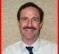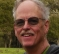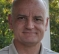## concept of bandwidthStarted by 4 years ago25 replieslatest reply 4 years ago415 views

I am trying to understand the concept of bandwidth from a point of view of bandwidth occupied by a signal.

Let me take an example to state my question.

Let us assume that there is a data source. The data source provides data at a rate as requested by a sampling block. The data is nothing but a sequence of random numbers. The max size of the numbers, I assume, does not matter but let us assume it is 8 bits.

The sampled data then go to a modulator that modulate the data as per some modulation scheme (modulation scheme does not matter) and transmits out.

Let us assume that the sampling block reads the data at the rate of S1 and S2. S2 being twice S1 (S2 = 2 * S1). Let us additionally assume that S1 and S2 are sufficiently high.

When I observe the bandwidth in frequency domain, based on S1 and S2, the bandwidth is same in both the cases.

Similarly, the value of the numbers does not matter in the sense that instead of 1, 4, 5 ..., I can have 2, 8, 10 and the bandwidth would be the same.

[ - ]Bandwidth is a property of the data being transmitted.  The sampling rate, as long as it exceeds the minimal Nyquist rate, has no effect on the bandwidth.  Data are sampled merely to reconstruct them and once reconstructed the bandwidth is independent of the sampling rate.The things you don't care about, the type of modulation and the data depth, do affect the bandwidth.

Greg

[ - ]Thanks, Greg.

so, instead of having 1,2,3,4 if I have 2,4,6,8 numbers, you mean to say the bandwidth occupied by such a signal will change. I thought, only the power varies.

[ - ]Sharan,

What I meant was if you represent a number with more bits then you need more bandwidth.  The actual values don't matter but the value 1 in an 8-bit number takes less bandwidth than the value 1 in a 16-bit number.

Greg

[ - ]Hi Greg,

Is it because you eventually transmit 8 bits in the former case vs 16 bits in the latter. The latter has 8 additional 0s even though both of them are equal to 1.

[ - ]Yes

[ - ]Hi Greg.

[ - ]Hi Rick,

I was referring to the amount of frequency needed to transmit the number in a fixed amount of time.

Greg

[ - ]Hi Greg. Ah ha, OK. Thanks.

[ - ]Hi Sharan, make your data complex with the real component being MAXVAL and your quadrature component being randomized. That will spread the signal across the entire spectrum of your sampling rate. (so sample at 20MHz get 20Mhz wide "noise", sample at 40MHz and get 40Mhz wide noise)

[ - ]Is your question related to the comments I left about bandwidth in the OFDM discussion the other day?

I may be wrong in my understanding of your question here, but I believe that the type of modulations has an incredible amount to do with the resulting bandwidth of a signal.  Your question here says that the sampling block pulls data at either S1 or S2 and that the resulting BW is the same.

Let's take a couple of examples of the possible modulations you could implement in the "sampling block".
1) Take every other sample and alternate using them as real and imaginary parts of a complex baseband signal and simply modulate using a complex carrier.  If S2 = 2*S1, then the resulting bandwidth for the S2 case is twice that of the S1 case.
2) Now consider an OFDM modulation scheme where the "sampling block" takes in the data you propose.  The sampling rate of the output signal can be adjusted, and the bits used in various ways can be applied so that the different sub-carriers have different numbers of bits per dimension, and the number of subcarriers within the band used in different ways, and you end up with MANY different results in terms of the bandwidth of the signal seen at the output of the D/A converter.

So, the rate of the input random data does not necessarily imply a direct relationship to the bandwidth of the resulting signal.

Let's review the history of various modulation schemes.

In the late '70s, the telephone bandwidth was almost completely used to send 300 bps.  Before we gave up on wired modems, we were sending 56 kbps over a more efficient use of only a little more than the same bandwidth.

[ - ]Hello dgshaw,

Regarding point 1 above.

let me take an example so that I am not confused again.

Let us take an array of data = [1,2,3,4,5,4,3,2,1,-1,-2,-3,-4,-5,-4,-3,-2,-1 ... repeat this sequence]

In case 1 of sampling, the samples are spaced with an period of t1. This is signal S1

in case 2 of sampling, the samples are spaced with an period of t2. This is signal S2

t2 = t1/2. That is, t2 period half of t1 or t2 frequency is double that of t1

So, the bandwidth of signal S2 is twice that of S1.

In other words, in any given time interval, we are packing more data bits for S2 compared to S2.

I hope my assumption is correct.

Regarding point 2 above,

I understand that modulation scheme has an impact on the overall bandwidth.

I want to bring up one more point which was raised in this post above.

In case of the signal defined above, if I use 16 bit samples instead of 8 bit samples, will it now increase bandwidth of the signal at the baseband?

For example, if I represent 1 as 0xb0000_0001 versus 0xb0000_0000_0000_0001

Hi Greg,

I apologize if my original question was not clear. The lack of clarity is due to my lack of understanding of this topic itself.

[ - ]Post deleted by author

[ - ]If your phrase "bandwidth" is related to the frequency-domain bandwidth of a time-domain sampled sequence, then the following is always true:

Let's say you sample an analog signal at an Fs1 sample rate (Fs1 is measured in samples/second) to produce the time-domain x1(n) sequence.

Next, you sample the same analog signal at an Fs2 sample rate (Fs2 > Fs1) to produce a different time-domain x2(n) sequence.

If Fs1 and Fs2 are both larger than twice your analog signal's bandwidth (measured in Hz), then the measured frequency-domain bandwidths (measured in Hz) of your x1(n) and x2(n) sequences will be the same!

Now, ...the number of bits used to represent the sample values in your two sampling processes will affect the background quantization noise of your two frequency-domain spectra. But the number of bits used in your two sampling processes will NOT affect the measured frequency-domain bandwidths of your x1(n) and x2(n) sequences.
[ - ]Hi Rick,

I would like to reverse the process and ask my follow-up questions ...

Assume, you have sampled an analog signal to produce time domain sequence x(n). Now I read out these samples in 2 ways:

1) samples are read out at the rate t1 time

2) samples are read out at the rate t2 time, where t1 = 2*t2

In both the cases above, I modulate and transmit.

what is the bandwidth occupied in the 2 cases above?

in case 2, we end up transmitting double the data that of case 1 within a given time interval

case 2, the frequency (if I convert digital data to analog), is double

[ - ]Hi Sharan123.

[ - ]Hello Rick,

In digital domain, where data to be transmitted is available then I have to readout or sample the data before doing final RF processing to transmit. Probably, data readout is not a correct term.

This is the clock frequency at which I sample the data for RF and transmission. I did not specifically use the term sampling to distinguish it from sampling that we normally do in analog domain.

[ - ]Hi Sharan123. We have a terminology problem here. In your Oct. 23 Comment, when you use the word "rate" I assume you mean "sample rate." And 'sample rates' are measured in samples/second. So when you wrote:

"samples are read out at the rate t1 time"

that confused me. "Rate" (sample rate) is NOT measured in seconds, so "rate = t1 seconds" makes no sense. Now if you said "The rate (sample rate) is such that the time between samples is t1 seconds." then that would make sense.

In any case, if your "rate t1" and "rate t2" are really the two sample rates of Fs1 and Fs2 samples/second, then my Oct. 23 Comment is still valid.

[ - ]Hello Rick,

I think I am focusing with the term and hence the actual issue is not being discussed.

I am copying part of your response below so that we can discuss further:

Let's say you sample an analog signal at an Fs1 sample rate (Fs1 is measured in samples/second) to produce the time-domain x1(n) sequence.

Next, you sample the same analog signal at an Fs2 sample rate (Fs2 > Fs1) to produce a different time-domain x2(n) sequence.

If Fs1 and Fs2 are both larger than twice your analog signal's bandwidth (measured in Hz), then the measured frequency-domain bandwidths (measured in Hz) of your x1(n) and x2(n) sequences will be the same!

I understand the explanation you have mentioned above. My confusion is not in this part.

Let us just take just x1(n) sequence alone.

Let us assume that we want to modulate this digital data and transmit on a wireless channel.

There are 2 cases to describe my question:

case 1

I send x1(n) samples for modulation at rate r1, say by sampling using a clock that has a period of r1.

case 2

I send x1(n) samples for modulation at rate r2

Here r2 = r1/2

So, in case 1, I end up sending complete x1(n) in total time window r1*N

Where N is the number of samples in x1(n)

In case 2, I end up sending complete x1(n) in time window r2*N

so, effectively, time window for case 2 is 1/2 time window for case 1

So, in case 2, I am sending data at a higher rate.

In these 2 cases above, how the bandwidth occupied by these data transmission.

I also request you to look at the following query.

Specifically, the following paragraph kind of highlights the conceptual issue I am struggling with:

The more information (or the higher the frequency of signal) we encode on our 'single sine wave' the broader the width of spectrum we take up. In fact, this is the original meaning of 'bandwidth' - how much of the band a signal takes up due to the encoded information on it.
[ - ]Below is my summary of bit rate/BW relationship:

Generation of signal in digital domain(Tx):

QAM(FDM based): bits => symbols (DAC) => BW equal symbol rate in the channel, shaped directly by a shaping filter. Thus a bw of 10 MHz can pass 10 Msymb/s i.e. 20 MBit/sec if QPSK

QAM(OFDM based, 15KHz spacing): asuming target of 10MHz(LTE) minus guard => 9MHz

bw controlled by number of subcarriers (600 in this case).

assuming all ofdm symbols carry data bits and bits mapped to data symbols using QPSK (not true) then bit rate = 600 * 2 per ofdm symbol = 600*2*140*100 = 16.8 MBit/s

Receiving analogue signal(Rx): Rx ADC does not necessarily need to know Tx sampling rate. Expects analogue signal of 10MHz BW at some frequency centre. shifts it to dc. This BW is inherent and should be faithfully passed to digital domain.

when it comes to digital generation of tones e.g. from look up table then frequency = sampling rate/number of samples per tone cycle. Once generated it can be upsampled and is expected to stay at same frequency. Though it can be shifted anywhere withing digital limitations.

[ - ]Hi Sharan123.
When you write:

"...for modulation at rate r1"

I have no idea what you mean. No idea at all.

I think you may be confusing the two concepts of "spectral bandwidth" and the terribly confusing phrase "transmission bandwidth." To avoid confusion, the word "bandwidth" should NEVER be used when discussing the "data transfer rate" (bits/second or symbols/second) of a communication system.

Let's say you have an anlog signal with spectral energy over the fequency of zero Hz to 50 Hz. That signal's bandwidth is 50 Hz. Now if you sample that analog signal at a sample rate of 2000 samples/second for one second you'll produce an x1(n) sequence having the following parameters:

CASE# 1:
Sample rate of Fs1 = 2000 samples/second
Bandwidth = 50 Hz
Time duration = 1 second
Number of samples = 2000.

Now if you sample that analog signal at a sample rate of 4000 samples/second for one second you'll produce an x2(n) sequence having the following parameters:

CASE# 2:
Sample rate of Fs2 = 4000 samples/second
Bandwidth = 50 Hz
Time duration = 1 second
Number of samples = 4000.

As for modulation: in Case#1 if you want to modulate a carrier sequence by the x1(n) sequence then the sample rate of the carrier sequence MUST be Fs1 samples/second. For CASE# 2, if you want to modulate a carrier sequence by the x2(n) sequence then the sample rate of the carrier sequence MUST be Fs2 samples/second.

So the whole idea of "sending x1(n) samples for modulation at rate r1" or "sending x1(n) samples for modulation at rate r2" makes no sense. Again, the sample rate of a carrier sequence must equal the sample rate of your x1(n) modulation sequence.

As for "What is the bandwidth of a modulated signal?", the "spectral bandwidth" of a modulated signal depends of the modulation type. For example, for a given modulating signal bandwidth (say, 50 Hz) FM modulated signals are wider in bandwidth than AM modulated signals.
[ - ]Thanks Rick.

I agree completely.  The bandwidth of the signal is not changed based on the number of bits per sample.  Only the signal to quantization noise ratio changes.

[ - ]Hi Rick,

May I add this interruption regarding confusing terminology:

In digital communication systems there are three sub-domains:

data bits, symbols, samples for DAC/ADC

The level of bitwidth has to be defined accordingly by OP.

[ - ]Hi Sharan123.

[ - ]Hello Rick,

I often get confused with the concept of bandwidth of a signal.

Further, I also get confused when it comes to data transmission as the data exists as digital information and at this point it is just a data and no corresponding signal exists.

Similarly, I don't know if signal shape impacts signal bandwidth. I assumed it does not but then as Greg says, if my amplitude of the signal is high then I need to represent these using more bits and hence I am effectively transmitting more bits.

Anyway, this is an academic question and not based on any issue I am facing.

[ - ]A starting point is to consider QPSK case

data bits are mapped to symbols, every two bits mapped to one symbol as below example:

00 => I/Q of 00, 01 => I/Q of 01, 10 => I/Q of 10, 11 => I/Q of 11

or you can map it your way..

symbols are then sent through DAC as +/- (peaks and dips).

Without shaping filter you will occupy wide band and get in trouble.

you shape it to narrowest useful band based  on this simple principle:

if you can pass frequencies from 0 up to half symbol rate you are ok since

+1 and -1 is the fast case of symbol transition and is passed. Anything higher is filtered off.(at edge of band a peak of tone at half symbol rate carries +1 and dip carries -1). Finally in the channel the bandwidth is doubled to equal symbol rate.